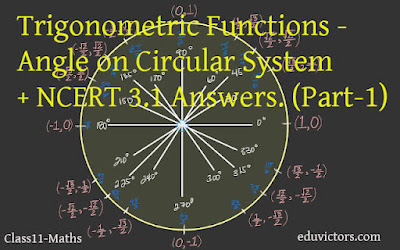# Class 11 - Maths - Trigonometric Functions - Angle on Circular System + NCERT 3.1 Answers. (Part-1)

The phrase "measuring the sides of a triangle" is how the word "trigonometry" is obtained from the Greek words "trigon" and "metron." The field was initially created to address triangle-related geometric issues. The surveyors who mapped out the new territories, the engineers, and other people studied it for navigation. Currently, numerous fields, including the science of seismology, the design of electrical circuits, the description of the condition of an atom, forecasting ocean tide heights, analysing musical tones, and many other things.

You should remember

1. In the sexagesimal system, we measure angles in degrees, minutes and seconds.

1 right angle = 90°

1° = 60′  (minute) and

1′ = 60′′ (second)

2. In the circular measure, we measure angles in radians.

Thus,

πc = 180°

3. If an arc of length l makes an angle $θ^c$ at the centre of a circle of radius r, we have

$θ = \frac{l}{r}$

4. A radian is a constant angle

5. If the length of an arc is equal to the circle's radius, then the angle subtended at the circle's centre will be one radian. One radian is denoted by $1^c$ and $1^c$ = 57° 16′ 22′′ c approximately.

Q1. Find the radian measures corresponding to the following degree measures:

(i) 25° (ii) – 47°30′ (iii) 240° (iv) 520°

(i) 25°

25° = $\frac{\pi}{180} \times 25 = \frac{5\pi}{36}$ radians

(ii) – 47°30′

= $–(47° + \frac{1}{2}°) = \frac{-95}{2}$

= $\frac{-95}{2} \times \frac{\pi}{180}$

= $\frac{-19\pi}{72}$

(iii) 240°

= $240 \times \frac{\pi}{180}$

= $\frac{4\pi}{3}$

(iv) 520°

= $520 \times \frac{\pi}{180}$

= $\frac{26\pi}{9}$

Q2. Find the degree measures corresponding to the following radian measures. (Use $\pi = \frac{22}{7}$)

(i) $\frac{11}{16}$

(ii) -4

(iii) $\frac{5\pi}{3}$

(iv) $\frac{7\pi}{6}$

(i) $\frac{11}{16}$

= $\frac{11}{16} \times \frac{180}{\pi}$

= $\frac{11}{16} \times \frac{180}{\frac{22}{7}}$

= $\frac{11}{16} \times \frac{180 \times 7}{22}$

= $\frac{315}{8}$

= $39\frac{3}{8}$

= 39°22′30′′

(ii) -4

= $-4 \times \frac{180}{\pi}$

= $-4 \times \frac{180}{\frac{22}{7}}$

= $-4 \times \times \frac{180 \times 7}{22}$

= $-\frac{2520°}{11}$

(iii) $\frac{5\pi}{3}$

= $\frac{5\pi}{3} \times \frac{180}{\pi}$

= 300°

(iv) $\frac{7\pi}{6}$

= $\frac{7\pi}{6} \times \frac{180}{\pi}$

= 210°

Q3. A wheel makes 360 revolutions in one minute. Through how many radians does it turn in one second?

Answer: No. of revolutions made by the wheel in 1 minute (60 secs) = 360

No. of revolutions in 1 second = 360/60 = 6

The wheel turns at an angle of 2π radian in one complete revolution.

In 6 complete revolutions, it will turn an angle of =  6 × 2π  = 12 π radian

Thus, in 1s, the wheel turns at an angle of 12π radian.

Q4. Find the degree measure of the angle subtended at the centre of a circle of radius 100 cm by an arc of length 22 cm.  (Use $\pi = \frac{22}{7}$)

l = 22 cm

θ = $\frac{l}{r}$ radians

θ = $\frac{22}{100} \times \frac{180}{π}$ degrees

θ = $\frac{126°}{10}$ = 12°26′

Q5. In a circle of diameter 40 cm, the length of a chord is 20 cm. Find the length of the minor arc of the chord.

r = 20cm

Thus the two radii and the chord forms an equilateral triangle.

∴  θ = 60° = $\frac{π}{3}$

l = rθ = $20 \times \frac{π}{3}$

l = $20\frac{π}{3}$

Q6. If in two circles, arcs of the same length subtend angles 60° and 75° at the centre, find the ratio of their radii.

Answer: Given, θ₁ = 60° and θ₂ = 75°

Let r₁ and r₂ be the two radii.

Since arc length(l) is equal and l = rθ

∴ r₁θ₁ = r₂θ₂

∴ $\frac{r₁}{r₂} = \frac{θ₂}{θ₁}$

= $\frac{75}{60} = \frac{5}{4}$

Thus the ratio is 5:4

Q7. Find the angle in radian through which a pendulum swings if its length is 75 cm and the tip describes an arc of length
(i) 10 cm
(ii) 15 cm
(iii) 21 cm

Answer: Since θ = $\frac{l}{r}$ radian
(i) l = 10 cm, r = 75 cm
θ = $\frac{10}{75}$ radian
θ = $\frac{2}{15}$ radian

(ii) l = 15 cm, r = 75cm
θ = $\frac{15}{75}$ radian
θ = $\frac{1}{5}$ radian

(iii) l = 21 cm, r = 75cm
θ = $\frac{21}{75}$ radian
θ = $\frac{7}{25}$ radian